# How to Rotate a Matrix in Ruby

Here is a problem that I recently stumbled upon while researching something completely different, as these things usually go, – and down the rabbit hole I went.

How does one rotate a matrix?

First of all, let’s define parameters of the exercise. We will take a matrix – an array of arrays – and turn it 90° clockwise.

So this is what it would look like, using Flatiron’s whiteboard table tops (we are really a paper-free school):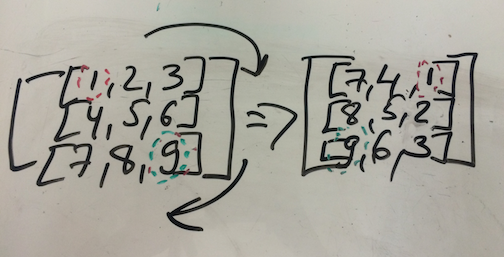As you can see, numbers 1 (position in zero-indexed terms) and 9 (position ) traded places. One thing is immediately obvious: this would only work if the matrix in question is ‘square’: that is, if there are x many rows with x many elements each.

Now that we have defined that condition, let’s start with a simple 3x3 matrix.

If the original array looks like this, Ruby-style:

my_array = [[1, 2, 3], [4, 5, 6], [7, 8, 9]]

The modified array would look like this:

rotated_array = [[7, 4, 1], [8, 5, 2], [9, 6, 3]]

But how to get there?

Ruby actually comes with default methods that allow one to manipulate arrays. Most people know pop, shift, unshift, and drop, but there are way more useful methods.

I was only marginally familiar with rotate, so here is an example:

According to the docs:

Returns a new array by rotating self so that the element at count is the first element of the new array.

When using it without an argument (or rather with the default count of 1), it works like this: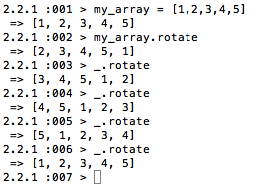It effectively takes the first part of the array and places it at the end of the array. If we use that 5 times on the array with 5 elements, we will arrive at the original array (well, it will return what looks like the original array as the original array does not get modified).

If used with a count that is not 1, it will move the elements down by the count number of places:(array.rotate(2) is the same as array.rotate.rotate)

Count can also be negative, in which case it will rotate the array in the opposite direction, taking items from the end of the array and placing them in the front: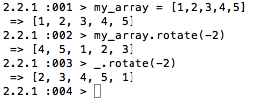As many other methods, it can be used with the bang to modify the original array: return!

I played around with rotate, and it became clear that I was going to need something else to complete the task: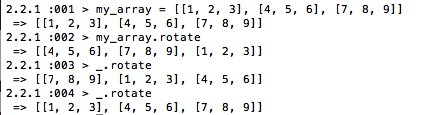It handily re-arranged the nested components in the array, moving each ‘inner’ array over by 1 at a time.

There is also transpose. It is actually a bit trickier to comprehend:For a nested array that contains 2 array of 2 elements each, it seems like all it does is switch elements that are in the  and  position with each other – in case below, only 2 and 3 traded places. But what is actually does, as becomes more obvious in case of a larger array (nexted with 3 arrays of 3 elements each) is make columns rows and rows columns.

Here is an example: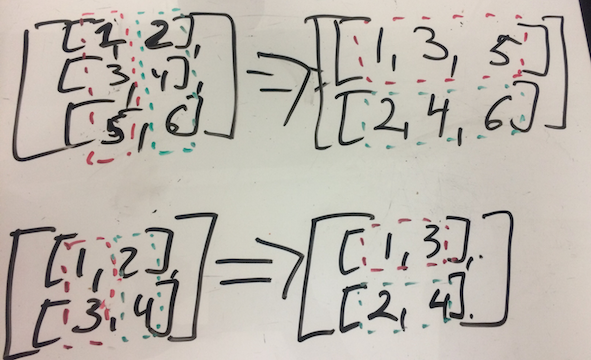Unlike rotate, transpose does not take any arguments.

By the way, although I won’t be using it here, one of my favorite array methods is also flatten, which is a handy way to get nesting out of arrays:As an aside, flatten is is also a handy way of converting a hash into a simple array:But back to the task at hand:

Upon experimenting with rotate, flatten, and transpose, I think I came up with a pretty efficient technique using transpose and then reverse: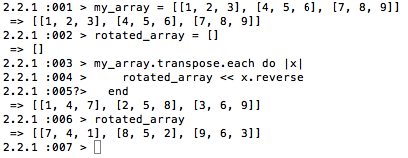The array is first transposed and then iterated over, and each of the nexted arrays inside it is reversed.

Works like a charm. But if you want to do something slightly more exciting:

Let’s look at the origin array again:

my_array = [[1, 2, 3], [4, 5, 6], [7, 8, 9]]

And at the rotated_array:

rotated_array = [[7, 4, 1], [8, 5, 2], [9, 6, 3]]

For a zero-indexed language, the nested array will look like this, positions-wise:

[6,3,0]
[7,4,1]
[8,5,2]

OR:

(position of first element (0 in this case) + 2*3) + (position of first element + 3*1 ) + (position of first element + 3*0) (position of second element + 2*3) + (position of first element + 3*1 ) + (position of second element + 3*0) (position of third element + 2*3) + (position of third element + 3*1 ) + (position of third element + 3*0)

Or for a nested array:

[,,],
[,,],
[,,]

Which is:

[[my_array.length-1][my_array.length-3],[my_array.length-2][my_array.length-3],[my_array.length-3][my_array.length-3]],
[[my_array.length-1][my_array.length-2],[my_array.length-2][my_array.length-2],[my_array.length-3][my_array.length-2]],
[[my_array.length-1][my_array.length-1],[my_array.length-2][my_array.length-1],[my_array.length-3][my_array.length-1]]

This would be a cool structure to use if pushing things into the array (I am using absolute positions instead of array.length-related as it is easier in this example):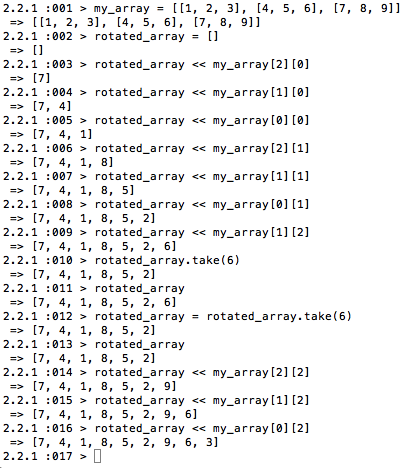(as you can see I made a typo and then fixed it)

All you need to do thereafter is convert it into a nested array.

You can also do a chain of push statements:All of these are pretty fun solutions to the problem.